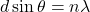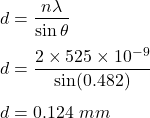Question

Light of wavelength 525 nm passes through a double slit, and makes its second maximum (m = 2) at an angle of 0.482 deg. What is the separation of the slits , d, IN MILLIMETERS ?

1.thachthao

d = 0.124 mm

Explanation:

It is given that,

Wavelength of light is 525 nm

It makes second maximum at an angle of 0.482 degrees

We need to find the separation of the slits.

For 2nd maximum, the equation for double slip experiment is given by :d = separation of the slitsSo, the separation of the slits is 0.124 mm.